DIY

# We measure high voltage with a multimeterWhen making homemade high-voltage structures, when adjusting the parameters of air ionizers, plasma lamps and other devices where a high power supply is used voltage, it becomes necessary to measure voltage in tens of kilovolts.
For this purpose, in professional activities, special devices are used – “kilovoltmeters”. Buying such a device deliberately makes sense only with its frequent use. But in the practice of a radio amateur, such measurements are not often performed. Therefore, to assess the parameters of the manufactured structure for high voltage, we can use a conventional multimeter, but with an additional attachment.
The upper limit of voltage measurement of a traditional multimeter, usually does not exceed 700 … 1000V. Therefore, to measure high voltage with a multimeter, it must be supplemented with an attachment, which will expand the measurement range.
Such a simple attachment – a kilovoltmeter for measuring voltages of 10 and more kilovolts, based on a voltage divider, we can make ourselves in the evening.
Voltage divider on resistors
Voltage divider is the simplest circuit that allows you to get a reduced voltage from high voltage using only two resistors. The output undervoltage will be a fraction of the input voltage, and will depend on the ratio of the resistance of the divider arms.The voltage divider circuit includes an input voltage source (V in) and two resistors R1 and R2. The voltage drop (Vout) across the resistor R2, this will be the undervoltage we need.
The voltage divider circuit is trivial, but such dividers are never used for heavy loads. The reason for this is the instability of the output voltage due to the influence of load resistance variables, temperature drift of the resistance of the resistors. The efficiency of such a scheme is low, a small part of the power reaches the load, and most of it is released on the resistors in the form of heat.
Relatively high voltages, but very small currents, function in the set-top box design; the set-top box is made in the form of a high-voltage and high-resistance voltage divider. This to a large extent allows to reduce the influence of the listed negative reasons and, with the correct calculation of the divider, to provide a real assessment of the controlled voltage.
Note that the load is connected to the voltage divider in parallel with the resistor R2, bypassing it. In this case, the total resistance of the divider arm decreases (recall the formula for parallel connection of resistances) and the voltage at the output of the divider changes. Therefore, in order to reduce the effect of the load, the resistance of the resistor R2 of the divider, it is advisable to set it much less than the resistance of the load, in our case, a multimeter with an internal resistance of 10 mOhm. (The internal resistance of simple multimeters is often 1 mΩ). This must be taken into account when calculating the voltage divider.
Calculation of the resistor voltage divider
In calculating the output voltage of the divider, the nominal values ​​from the above diagram are used. Knowing these values, we can calculate the output voltage using the following formula based on Ohm's Law.
V out = V in x R2/(R1 + R2)
In some cases, having specified the values ​​of the input and output voltages (Vin, Vout), it is necessary to calculate the resistance of the lower arm R2 for the existing high-resistance resistor R1. This will be the case in our example as well. Then we can calculate the required value of the resistor R2 using the following formula:
R2 = (R1 x Vout)/(Vin – Vout)
However, we can simplify the task by turning to the Internet, as there are many online calculators for quickly calculating the voltage divider.
Note, the ratio of voltages between R1 and R2 is determined only by their relative values. The values ​​of the arm resistors can vary, it is only important to maintain their ratio.
Making a voltage divider
We will make a two-range attachment to a multimeter for monitoring voltages up to 10 kilovolts, with a voltage divider across resistors. Voltage division factor “1000” – 1 measurement range, “100” – 2 range. This divider variation is based on the above basic scheme.
1. Schematic of the set-top box being manufactured: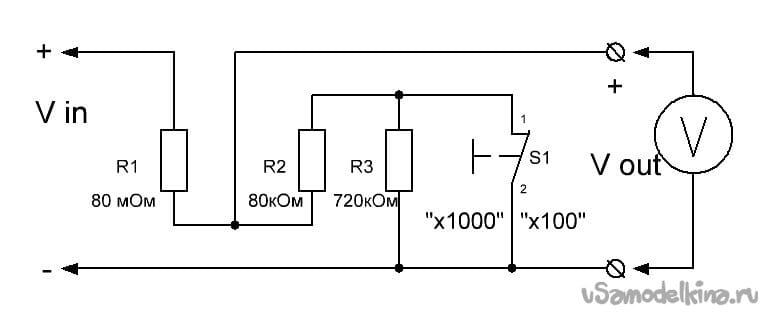Schematic diagram of an attachment for measuring high voltages (up to 10 kilovolt) using a multimeter. Resistor values ​​are approximate.
As the upper arm of the divider R1, a high-voltage resistor KEV-1 with a resistance rating of 100 mΩ is used. The actual resistance of the resistor is about 80 mΩ.
The lower arm of the divider R2 (upper basic circuit), in the attachment, consists of two series-connected resistors with a total resistance of about 800 kOhm.
In the normal position, the S1 button shunts one of the chain resistors (R3), in this position the arm resistance will be about 80 kΩ (the division factor is the ratio of the resistors R1 and R2, equal to 1000 – 1 measurement range). By pressing the S1 button, the arm resistance increases to 800 kOhm (the division factor will be equal to 100 – 2 range). The second measuring range is turned on to clarify the Vout reading of the multimeter V, at low values ​​of the input voltage Vin.
It should be noted that each of the resistances R2 and R3 in the set-top box circuit can be composed of two or three resistors. This is necessary to precisely match the calculated resistance value in both measuring ranges. For example, with the resistance of the upper arm of the divider R1 equal to 80.0 mΩ, the resistance of the lower arm R2 in 1 range, taking into account the load from the internal resistance of the multimeter 10 mΩ, should be equal to 80.645 kΩ, which has to be selected from several resistors.
2. Set-top box set
For the upper arm of the R1 divider, we use a permanent non-wire, high-voltage, varnish film, with a composite varnish conductive layer, a KEV-1 resistor for surface mounting. Resistors are intended for operation in electric circuits of direct and alternating currents.
Main technical characteristics of KEV-1 resistors:
– Range of nominal resistances: 510 kOhm … 47 gOhm
– Maximum allowable dissipated power – 0.5 ; one; 2; five; 10; 20 and 40 W
– Operating voltage from 2.5 to 60 kV (depending on power)
– Temperature coefficient of resistance in the range -60 … + 20 ° С – no more than 0.35%/°
– Permissible deviations of resistances: ± 5; ± 10; ± 20%
– Operating temperature -60 … + 100 ° С
In this example, we use a KEV-1 resistor of 100 mΩ, with a power of 1 W, operating voltage up to 10 kV. Most common resistors are rated for 200 V.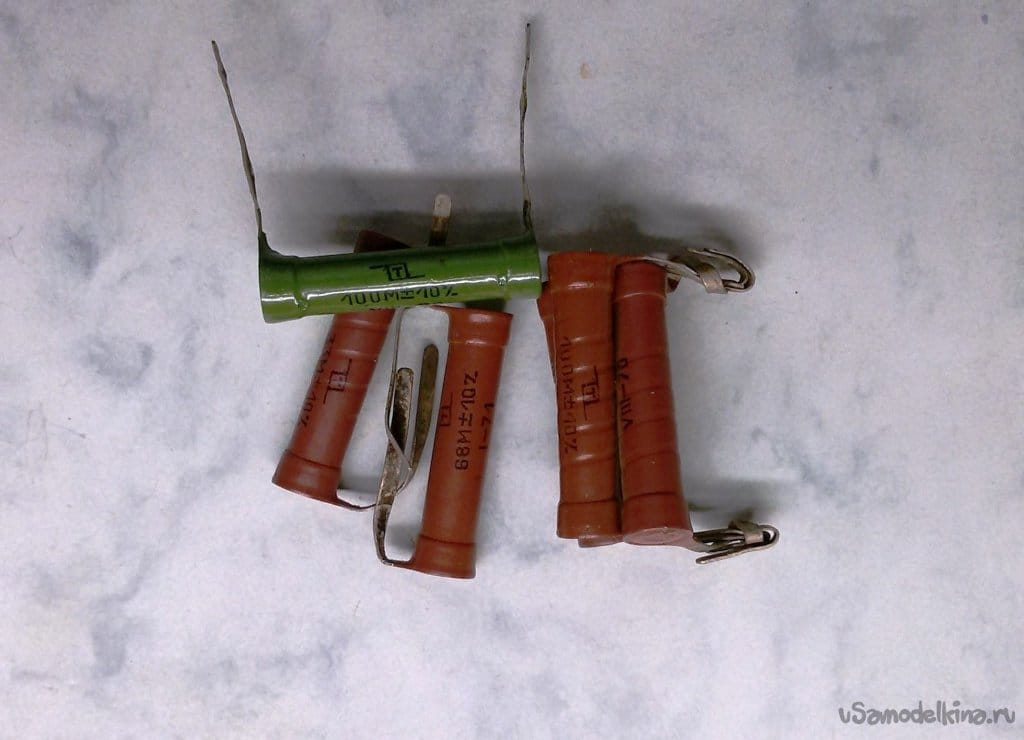The rest of the MLT-0.5 resistors, the D301 button with opening contacts, they will operate at voltages up to 80 – 100 V.
A plastic tube (from an instantaneous water heater) with a diameter of 20 mm and a length of 150 mm is used for the body. A stem from a medical syringe is used as a board for volumetric mounting of resistors. Let's adjust its diameter to fit tightly into the tube.
A measuring probe from an old device will serve as the tip of the attachment.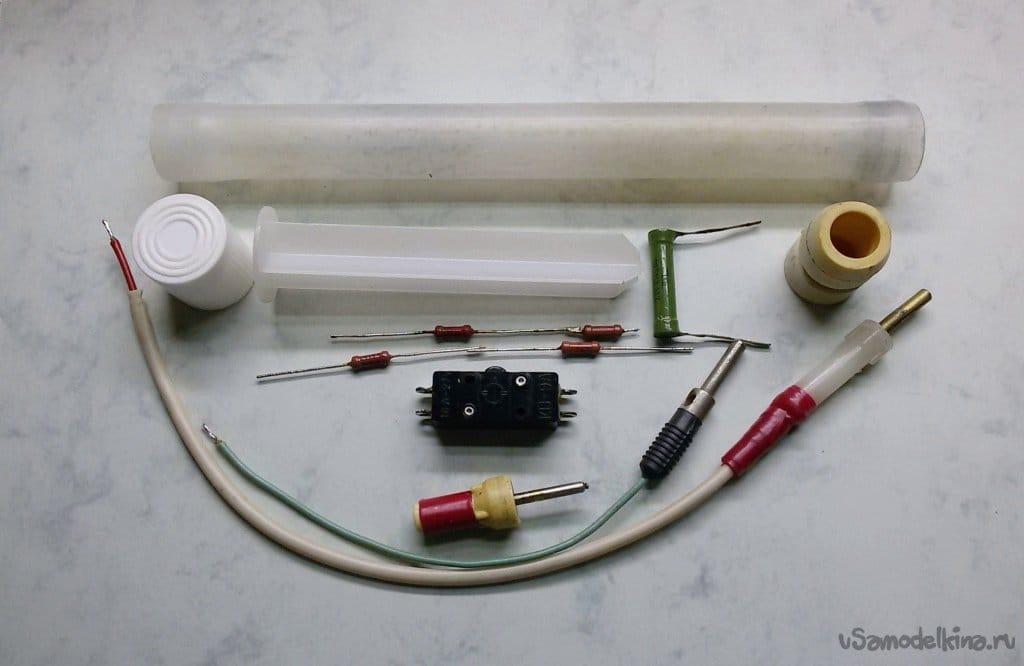3. Making attachments

With the threaded part of the probe we fix the tip in the stop of the syringe stem. Determine the position of the microswitch in the tube, it is determined by the length of the stem. According to the location of the switch, in the tube wall we will process a hole with a diameter of 8 mm to access the button.In the chambers between the ribs of the stem, we install a chain of resistors according to the attachment diagram. Plastic ribs serve as a base for mounting parts and an insulator that allows you to fold a long chain and shorten the length of the attachment. Installation must exclude the occurrence of leakage currents, the dielectric properties of the base must correspond to the level of the measured voltage.
We carry out the necessary connections and assemble the structure of the attachment in the case. The back of the tube is closed with a plastic cap of a suitable diameter.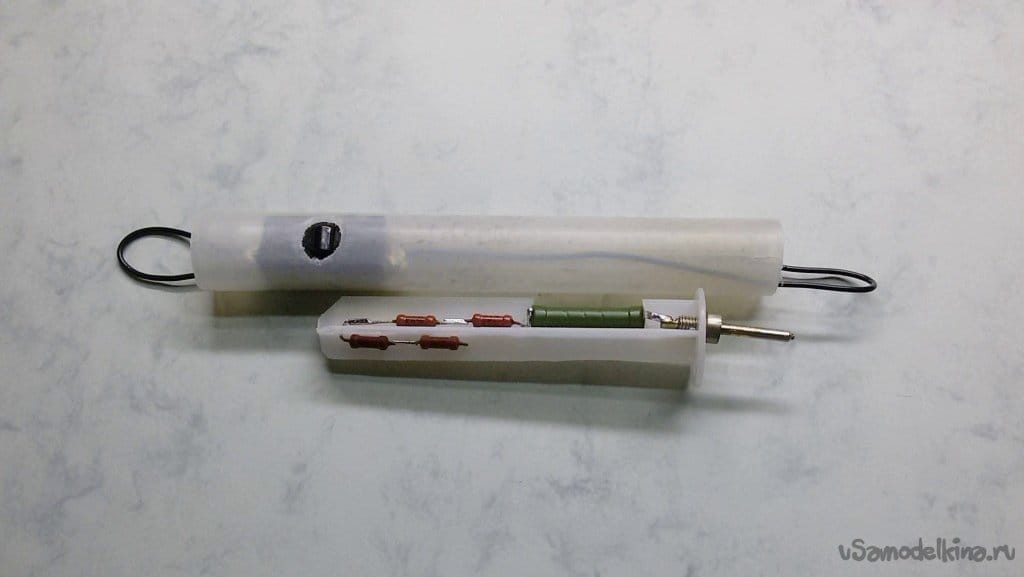4. Using a prefix

Black wire with reinforced insulation is common (-) for Vin and Vout. Since it is connected to a high-voltage circuit, the requirements for its insulation and electrical safety rules must be appropriate. This wire is taken out from the contacts of the button, in the middle of the case.
The low voltage part of the divider circuit is located at the rear of the case. The test lead (+) to the multimeter goes through the cap on the end of the case.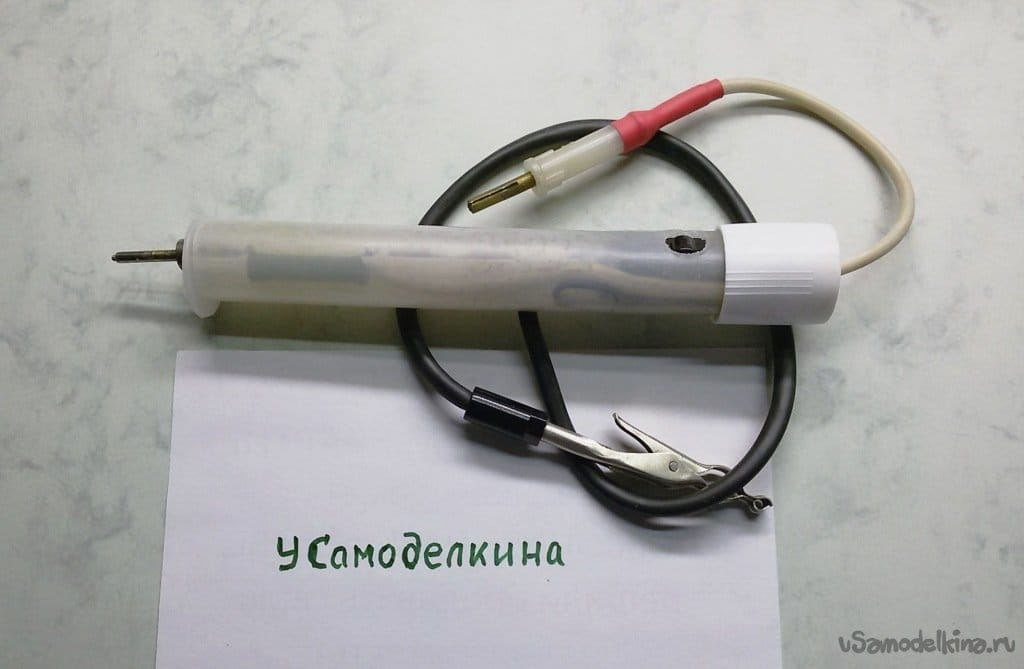To measure high voltage, we connect a multimeter in the range of 100… 200 V to the corresponding terminals of the attachment. We connect the common wire to the high-voltage device. We turn on the HV device.
With a measuring probe on the front side of the case, we touch the high voltage source.
We take readings of the device in 1 measuring range “1000”. At low voltage values ​​on the scale of the device, press the button and switch the prefix to the range 2 “100”.
5. Testing the set-top box
Let's check the operation of the set-top box on alternating current in the 220V network.
The tester connected to the network shows exactly 220V.Switch the multimeter to the console. 220/1000 = 0.22.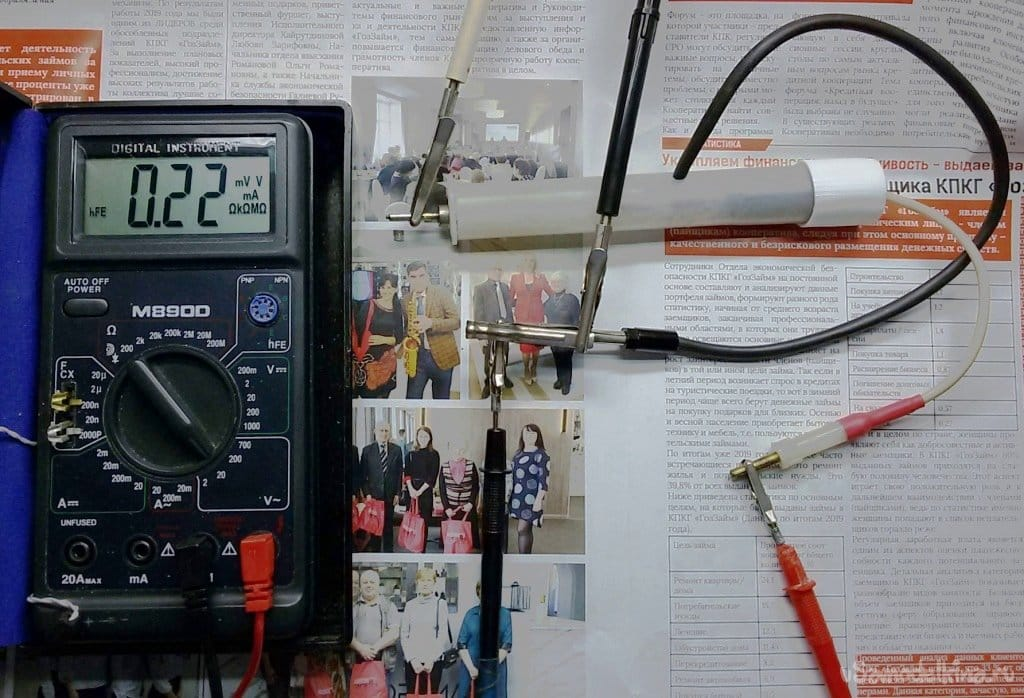Press the button on prefix, the readings increased by about 10 times. Inaccuracy is caused by voltage fluctuations in the network, inaccurate calculation or selection of resistors. Further practice will show.Let's move on to high voltage and check the newly manufactured transformer.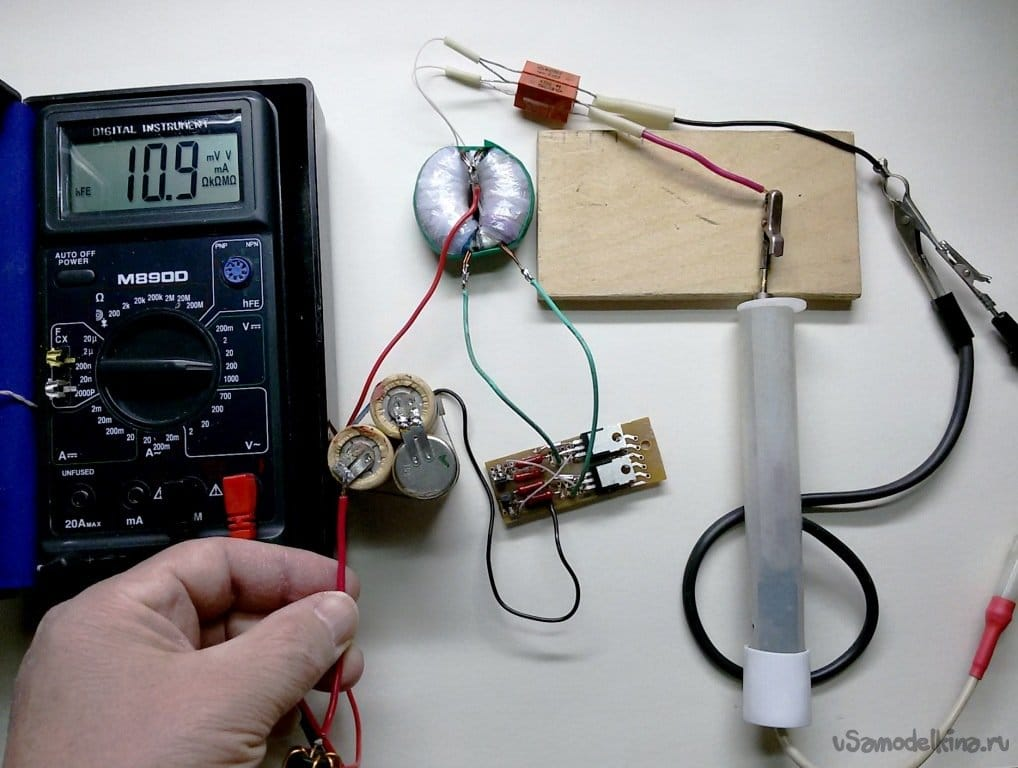Similar measurements show that the rectified RF voltage is about 10 kV and that it is necessary to more accurately select the resistance of the lower arm of the voltage divider.
If you need more accurate measurements of high voltage, you can send a signal from the set-top box to the oscilloscope, you will see the amplitude and shape of the pulses.
If you want to assemble the set-top box on a voltage divider with a factor of 10,000, you can assemble a chain of ten high-resistance resistors connected in series with 68 mOhm (the upper arm of the divider with a total resistance of 680 mOhm) and one resistor (lower arm) with a resistance of 68 kOhm. During installation, all resistors must be arranged evenly in a line, at a length of at least 200mm to prevent breakdown in the attachment.
6. Safety precautions
When using a kilovoltmeter, you should observe safety measures.
Connect and disconnect the device with de-energized equipment, after removing the charge from current-carrying high-voltage parts.
When connecting the device to the measured circuits, connect the grounding first of all.
When disconnecting the probe from the measured circuits, disconnect the grounding last.

Source:

usamodelkina.ru

Check Also
Close